Up: No Title Previous: No Title

Example 1: Force on a sphere accelerating (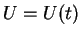, unsteady) in an unbounded fluid at rest. (at infinity)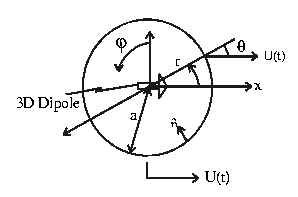K.B.C on sphere: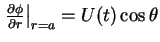Solution: Simply a 3D dipole (no stream)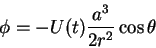Check:Hydrodynamic force: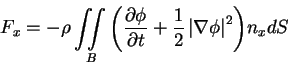On r = a: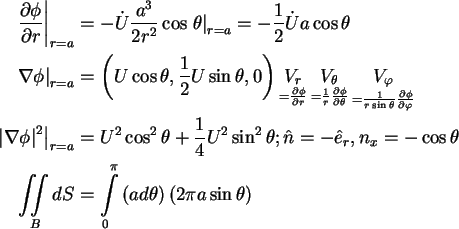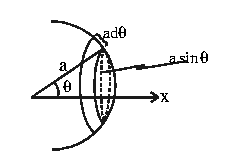Finally,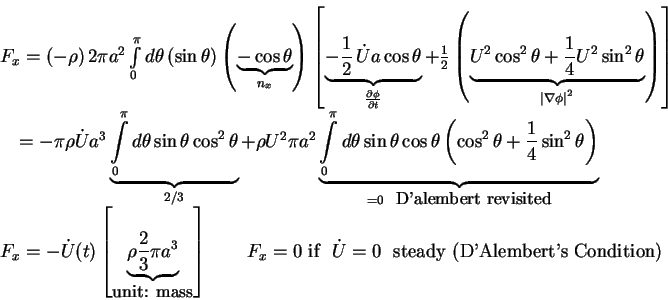General 6 degrees of freedom motions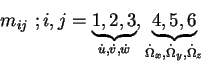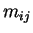: associated with force on body indirection due to unit acceleration in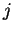direction. For example, for a sphere: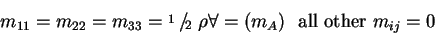Some added masses of simple 2D geometries
• circle figure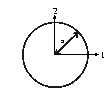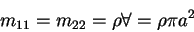• ellipse figure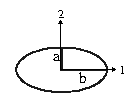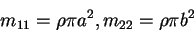• plate figure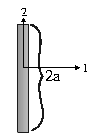• square figure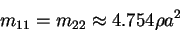A reasonable estimate for added mass od a 2D body is to use the displaced mass (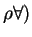of an equivalent cylinder'' of the same lateral dimension or one that rounds off'' the body. For example, we consider a aquare:
1.
inscribed circle: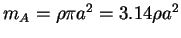.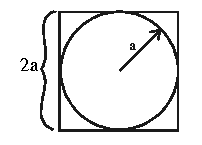2.
circumscribed circle: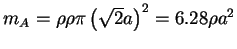.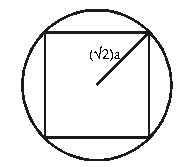Arithmetic mean of 1) + 2)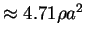.

General 6 degrees of freedom forces and moments on a rigid body moving
in an unbounded fluid ( at rest at infinity)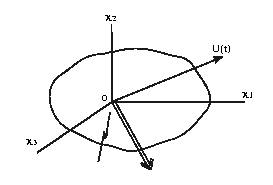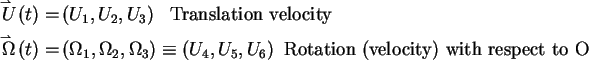Note:fixed in the body.

Then (JNN §4.13)
• forces

• moments

Einstein's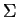notation applies.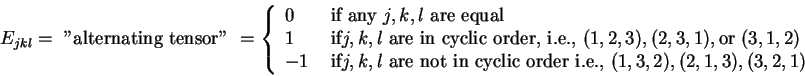Note:
1.
if0 ,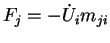(as expected by definition of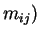. Also if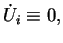then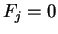for any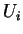, no force in steady translation.
2.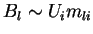added momentum'' due to rotation of axes, 2)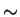where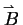is linear momentum. (momentum from 1 coordinate into new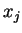direction)
3.
If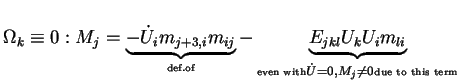.
Moment on a body due to pure steady translation - Munk'' moment.
Example of Munk Moment - a 2D submarine in steady translation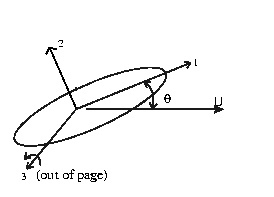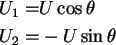Consider steady motion: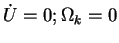. Then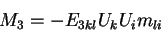For a 2D body,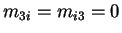, also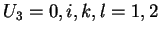. This implies that:

Therefore,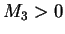for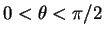("Bow up"). Therefore, a submarine under forward motion is unstable in pitch (yaw) (e.g., a small bow-up tends to grow with time), and control surfaces are needed: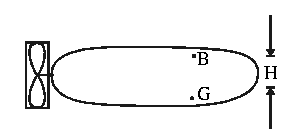• Restoring moment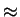(g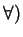Hsin.
• critical speed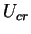given by: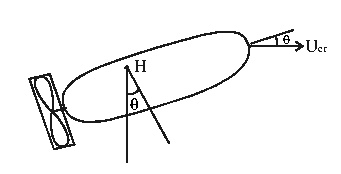Usually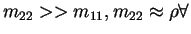. For small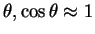. So,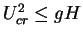or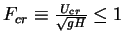. Otherwise, control fins are required.Up: No Title Previous: No Title